# Working with the String Class in Visual Basic .NET

### Working with the String Class in Visual Basic .NET

When you manipulate string objects, you'll use the methods that are part of the String class. There are two flavors of String class methods. They are

• Shared methods ” These are inherent in the String class itself. As such, they don't require an instance of the String class to function.

• Instance methods ” These methods are derived from the specific instance of a String object. This means that an instance method is always qualified with the name of the String object.

The distinction between these two method types will become clearer when we start using them. To begin our discussion of using the String class, let's explore how several of our earlier String functions can be duplicated with methods of the String class.

#### The Length Method

You already saw how we can calculate the length of a string using the Len() function. You can accomplish the same thing using the Length method of the String class. To test this method, remove all the lines from the btnCalc Click event and add the following new lines:

` Dim buff As String  buff = txtString1.Text txtResult.Text = CStr(buff.Length) `

First, we define a variable named buff of type String . Next , we move the string data from the txtString1 text box into buff using the assignment operator. Now look at the right side of the assignment statement in the third line. The expression

` buff.Length `

in essence is telling Visual Basic .NET: "Use the Length method of the String class to find how many characters are currently stored in the variable named buff ." Note that the dot operator is used to separate the instance name of the string variable from its method name.

Because the Length method requires us to specify the name of the variable being used, Length is an instance method. Recall that instance is an OOP term that refers to a variable that has been properly defined, often with a Dim statement. In our example, instance means that we have a variable named buff that exists in memory (that is, it has an lvalue ) that we can use in our program.

Figure 6.9 shows a sample run. As you would expect, it yields the same results as does the Len() function.

##### Figure 6.9. Sample run using the Length method of the String class.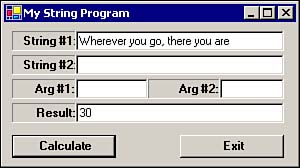Notice that the output in Figure 6.9 is identical to that shown in Figure 6.3. So far, so good.

#### The Concat Method

String concatenation is very simple. Remove the current lines from the btnCalc Click event and add the following line:

` txtResult.Text = String.Concat(txtString1.Text, txtString2.Text) `

String concatenation using the String class Concat method simply uses a comma-separated list of the strings you want to concatenate together. A sample run is shown in Figure 6.10, using the same data we used for Figure 6.2. It should come as no surprise that the results are identical. (Don't forget to add the trailing blank space after Joyce .)

##### Figure 6.10. Sample run using the Concat method.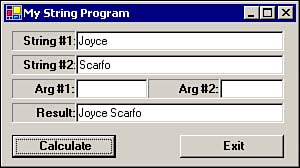Note that the Concat method is not prefaced with the name of a variable. Instead of a string variable name, it's prefaced with the keyword String . After the String keyword comes the dot operator, the Concat method name, and finally the parenthesized list of strings to be concatenated . Because the method is prefaced with the String class name, the Concat method is an example of a shared String method.

Programmer's TipThe Concat method is not limited to concatenating two strings at a time. You can concatenate as many strings as you want. Simply place them in order, going from left to right, and separate each one with a comma. For example ` SubStr = String.Concat(Str1, Str2, Str3, Str4, Str5) ` would concatenate five strings together and assign the result into SubStr .

##### Optimizing String Concatenation

String data is sometimes used to store data in rather strange ways. For example, I was involved with a project that had to track each unique word as it appeared in a database of medical terms. This required reading the database and appending each new term to a string, followed by a comma. On completion of reading the database, the (rather large!) string contained a comma-separated list of all the unique (17,000+) medical terms in the database.

When the program was written, the string concatenation statement looked like

` MedicalTerms = MedicalTerms & NewTerm & "," `

The program execution was extremely slow. A little digging around showed that a surprising amount of the execution time was being spent on the concatenation statement. We scratched our heads for a few minutes and then had a flat-forehead epiphany: Strings in Visual Basic .NET are immutable, which means you can't change them after you create them. This also means that, as the program executed, Visual Basic .NET was passing 17,000+ messages back and forth to Windows asking for storage for a new (longer) string.

We changed the code with the following modifications:

` Dim TempStr As New System.Text.StringBuilder(255000)  Dim MedicalTerms As String, Term as string                    ' Some details left out...   TempStr.Append(Term)   TempStr.Append(",")                    ' More details left out... MedicalTerms = TempStr.ToString `

The key change is the definition of the TempStr object, which is an instance of a StringBuilder() object. We defined the object to be capable of holding 255,000 characters, or about 15 characters per term. The code uses the Append() method of the StringBuilder() object to build the string. (The Append() method statements are actually inside a program loop; a topic we'll discuss in Chapter 12, "The For Loop.") The final statement of TempStr into MedicalTerms is necessary because the StringBuilder() object is different from a String data type.

What did we gain from the program changes? The actual execution speed was approximately three times faster than before. However, the execution speed of the loop that built the string of terms was almost 30 times faster than before. Clearly, the StringBuilder() object is optimized by Visual Basic .NET to perform string concatenation and minimizes message passing between Visual Basic .NET and Windows.

#### The SubString Method

The SubString method has functionality that's similar to the Mid() function we studied earlier in this chapter. To experiment with this method, remove the current lines from the btnCalc Click event and add the following lines:

` Dim buff As String  buff = txtString1.Text txtResult.Text = buff.SubString(CInt(txtArg1.Text), CInt(txtArg2.Text)) `

Note that the SubString method is prefaced with the name of the variable we want to use. Therefore, SubString must be an instance method. The first argument of the method (that is, txtArg1.Text ) is the starting position for the substring. The second argument of the method ( txtArg2.Text ) is the number of characters, or length, we want to extract. Because these two arguments are stored in text boxes, we use the CInt() conversion routines to change them from String data to numeric data, which is the data type the SubString method expects for both arguments.

Figure 6.11 shows a sample run of the SubString method. The sample run uses the same data that we used for the sample run shown in Figure 6.6. Oh-oh the results aren't the same. What's the problem?

##### Figure 6.11. Sample run using the SubString method.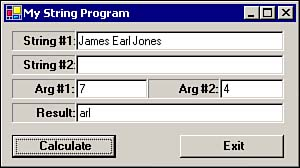##### Character Counting with the String Class

Well, it's really not a problem. It's just the old off-by-one syndrome. The reason that the results aren't the same is because all String class methods treat the first character in the string as character number 0. On the other hand, built-in Visual Basic .NET functions such as Mid() , treat the first character in the string as character 1. In the string James Earl Jones , if you treat the first J as character 1, E becomes the seventh character. However, if the first J is character 0, the a becomes the seventh character.

The lesson is simple: If you use the built-in Visual Basic .NET string manipulation functions, characters are counted from left to right, starting with 1 for the first character. You'll often hear these referred to as one-based calculations. If you're using the String class methods for string manipulation, characters are counted from left to right, starting with 0 for the first character. The String class methods are often referred to as zero-based calculations.

So, how do we modify our sample program run to produce the same results? Well, because String class methods are zero-based operations, we need to subtract one from our starting position. This is shown in Figure 6.12. (Don't forget the 4 for the second argument.)

##### Figure 6.12. Sample run of the Substring method, accounting for zero-based calculations.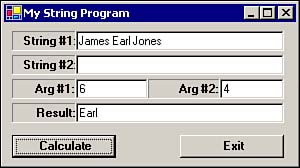Now the results in Figure 6.12 agree with those shown in Figure 6.6.

#### Emulating Left() and Right() Using the SubString Method

There are no String class methods that match the Left() and Right() functions directly. However, it's easy enough to emulate them using the SubString method. For example, suppose that we want to emulate the Left() string function. Remove the current lines from the btnCalc Click event and add the following line:

` Dim buff As String  buff = txtString1.Text txtResult.Text = buff.SubString(0, CInt(txtArg1.Text)) `

So, what does this program statement do? It says that we want to extract a substring that starts at position 0 with a length that equals the number held in the txtArg1 text box. This is the same thing that the Left() function does. A sample run of the new code is shown in Figure 6.13, using the same string used in Figure 6.4. As expected, the results are the same.

##### Figure 6.13. Emulating the Left() function with the SubString method.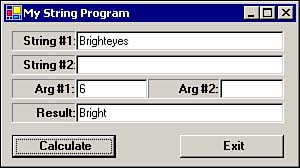Emulating the Right() function is similar, but takes a little more code to accomplish. Add the following code to the btnCalc Click event:

` Dim buff As String  Dim SubLength As Integer, SubStart As Integer buff = txtString1.Text SubLength = CInt(txtArg1.Text)     ' Get substring length SubStart = buff.Length - SubLength   ' Where to start txtResult.Text = buff.Substring(SubStart, SubLength) `

First, notice that we've defined two new variables in the btnCalc Click event. As you can see from the preceding code, SubLength is an integer variable that we use to hold the number of characters we want for the new substring. We get this number by converting the content of the txtArg1 text box into an integer using our old friend CInt() .

The next line determines the starting position in the string from which the substring is to be extracted. For example, if the string is Bluebird and we want to extract bird as the substring, the calculation becomes

` SubStart = buff.Length - SubLength  SubStart = 8 - 4 SubStart = 4 `

Plugging this information into the final expression finds

` txtResult.Text = buff.Substring(SubStart, SubLength)  txtResult.Text = buff.Substring(4, 4) `

Because String class operations are zero-based, character position 4 is the b in the string Bluebird , so the substring becomes the rightmost four characters, yielding txtResult.Text being assigned the substring bird .

#### Searching for Substrings

The String class provides the IndexOf method for finding the starting position of a substring in a way that is similar to the InStr() function we examined earlier. The syntax for the IndexOf method is

`  StartPosition  =  TheStringToSearch.  IndexOf(  SubStringToFind  ) `

To implement this method, remove the existing code from the btnCalc Click event and add

` Dim buff As String  Dim SubStart As Integer buff = txtString1.Text SubStart = buff.IndexOf(txtArg1.Text) txtResult.Text = CStr(SubStart) `

The real work is done by the program statement in the fourth line. The IndexOf method searches the string named buff for the substring held in the txtArg1 text box. If a match is found, the IndexOf method returns an integer that corresponds to the character position where the match occurred. If no match is found, a value of 0 is returned by the IndexOf method.

Note that we don't have to convert the value returned by the IndexOf method to an integer using the CInt() conversion routine. The reason is because Visual Basic .NET already knows that the IndexOf method returns an integer value, so a conversion is unnecessary.

Figure 6.14 shows a sample run using the same inputs as shown in Figure 6.8. Recall that we were trying to find the blank space between the first and last name. (There's a blank space in the txtArg1 text box used for the search string even though we can't see it.) Once again, the results in Figure 6.14 differ from those shown in Figure 6.8. The reason for the difference is because the current program uses a zero-based count, whereas Figure 6.8 uses a one-based count for the character positions .

##### Figure 6.14. Sample run using the IndexOf method.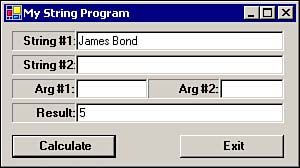##### The LastIndexOf Method

The LastIndexOf method is a useful variation of the IndexOf method. To illustrate , suppose that you have some information in a string that contains the city, state, and ZIP Code all packed into a single string:

` "Mt. Holly, NJ 08060" `

Let's further suppose that you want to extract the ZIP Code as a substring. There are several ways to do it. One way would be to use the Right() function and extract the five rightmost characters. Good plan, until someone slips in one of those nine-digit ZIP Codes we're supposed to be using.

Another way would be to search for a blank space between the state and the ZIP Code. The problem here is that there are two spaces in the string (that is, between the period and the H and between the comma and the N) before we get to the space we need. Hmmm

Not a problem. Simply use the LastIndexOf method. This method works much the same way as the IndexOf method, but the search starts at the end of the string and works backward toward the start of the string looking for a match. In other words, if you had the following code

` buff = "Mt. Holly, NJ 08060"  SubStart = buff.LastIndexOf(" ") `

SubStart would equal 13 , which is the zero-based character position of the last blank space in the string.

#### Finding Out What Is Stored at a Given String Position ”The Chars Method

Sometimes you'll find that it's more efficient to use string data to encode information. For example, suppose that you want to store the information about a college student. Let's further assume that class standing is encoded as 1 for a freshman, 2 for a sophomore, 3 for a junior, 4 for a senior, 5 for a master's student, and 6 for a Ph.D. student. Now let's assume that 0 is used to represent a female student and 1 is a male student. Let's also assume that computer science majors are encoded as a 7, and college of business majors are an 8. Finally, we'll assume that the student's class standing is stored in position 0 of a string, the student's sex is in position 1, and their major is stored in position 2. Therefore, the string

` "407" `

tells us that this student is a female student in her senior year majoring in computer science. That's quite a bit of information about the student stored in only three bytes of data.

Note that we could have created three separate variables to store this information. In this case, we've decided to trade off clarity for compactness. That is, it isn't intuitively obvious that the string 407 contains all the information that we've encoded into it. Because the data is encoded, we need to unpack the information from the string if we want to use the information contained in it. We can use the Chars method to help us extract the information.

The syntax for the Chars method is

`  CharacterStoredThere  =  MyString.  Chars(  PositionToExamine  ) `

The Chars method has a single argument, which is an integer value that corresponds to the character position in MyString we want to examine. The Chars method then returns the character stored in that position as a character data type. (In the strictest sense, the Chars method does return a Char data type, but Visual Basic .NET is smart enough to promote it automatically to a String data type if you assign its return value into a string.)

Remove the program statements from the btnCalc Click event and add the following program statements:

` Dim buff As String  buff = txtString1.Text txtResult.Text = buff.Chars(CInt(txtArg1.Text)) `

The argument to the Chars method is the character position in the string you want to examine. Figure 6.15 shows a sample run.

##### Figure 6.15. Sample run using the Chars method.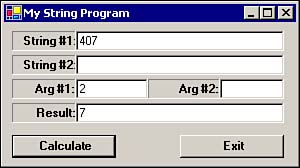In Figure 6.15, the value held in text box txtArg1 is the character position we want to examine; position 2, in this example. The result is shown in the txtResult text box, which is 7 . Again, while it looks as if the 7 is in position 3, the String class performs all of its operations using zero-based indexing. With that in mind, you can see that the Chars method returns the character stored at the position indicated.

#### Comparing Strings ”The Compare Method

A common task in a computer program is to compare one string against another string. For example, the user might type in the last name of a customer and then have the program search through a list of all customers trying to find a match to see whether the customer is a new or existing customer.

The Compare method enables you to compare two string values. The syntax for the Compare method is

`  Result  = String.Compare(  MyString, YourString  ) `

The Compare method compares MyString to YourString and returns an integer value to indicate the outcome of the comparison. If the value returned by Compare is 0, the two strings are identical. A non-zero value means the two strings are different. In most cases, that's all you need to know about using the Compare method. If you're into details, a positive return value means that MyString is greater than YourString . Think of this value as being determined by a subtraction of the Unicode values in the two strings. For more details, search the Visual Basic .NET online Help for "string comparison" for the index of the search. I'll provide additional details in a minute.

To use this method in our test program, remove the current program statements from the btnCalc Click event and add

` txtResult.Text = String.Compare(txtString1.Text, txtString2.Text) `

There are two things to notice in this program statement. First, this is a shared method of the String class. This is why the Compare method is prefixed with String . rather than the name of a string variable. The second thing to notice is that even though Compare returns a numeric value, Visual Basic .NET is smart enough to convert the numeric value to a string and then assign the result into the txtResult text box. (It wouldn't hurt anything, of course, if you enclosed everything on the right side of the equal sign in the parentheses of CStr() , thus converting the integer result to a string.)

Now let's examine some sample runs using the Compare method, as shown in Figure 6.16.

##### Figure 6.16. Sample run using the Compare method; unequal strings.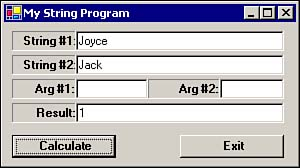In Figure 6.16, the two strings are different and the result is nonzero as expected. Why is the value a positive 1? If you look at the ASCII codes in Appendix A, you'll find that the value for a capital J is 74. Because both strings begin with a J, 74 minus 74 yields zero. So far, the strings match. Visual Basic .NET then examines the second character in each string and compares them. The o in Joyce is compared to the a in Jack. A lowercase o has an ASCII value of 111, whereas the letter a has an ASCII value of 97. Therefore, because 111 minus 97 produces a result that's greater than 0, the value 1 is returned from the Compare method.

Hmmm. If that's the case, the same logic should produce -1 if we reverse the two strings. This result is shown in Figure 6.17.

##### Figure 6.17. Sample comparison run, reversing the strings.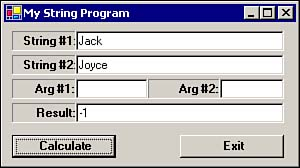Now try comparing JOYCE with joyce . This test is shown in Figure 6.18.

##### Figure 6.18. Sample comparison run, using uppercase versus lowercase letters in the strings.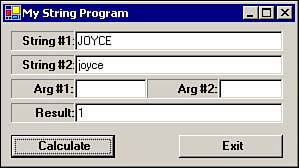If you look in Appendix A, you'll find that the uppercase J is 74, whereas the lowercase j is 106. If we do the math, 74 minus 106 is “32, which should yield a return value of “1, not a positive 1. Well, Visual Basic .NET throws us a curve here. When comparing strings with the Compare method, Visual Basic .NET scales uppercase letters so that they're numerically greater than lowercase letters. This explains why our other two sample runs performed as expected, but this example does not.

Programmer's TipRemember that uppercase letters are scaled to have values that are greater than their lowercase equivalents when used with the Compare method. This might save you some head-scratching later on.

#### The Insert String Method

There are times when you'll need to alter the content of a string variable. Although there are many alternative ways to accomplish such tasks , the Insert and Replace methods are perhaps the easiest to use. Let's look at the Insert method first.

Remove the current program statements from the btnCalc Click event and add

` Dim buff As String  Dim InsertPosition As Integer buff = txtString1.Text InsertPosition = CInt(txtArg1.Text) txtResult.Text = buff.Insert(InsertPosition, txtArg2.Text) `

In this example, the integer variable is used to tell Visual Basic .NET where to insert the new text into the string. The text to be inserted is held in the txtArg2 text box, while txtArg1 holds the character position for the insertion. Figure 6.19 shows a sample run.

##### Figure 6.19. Sample run using the Insert method.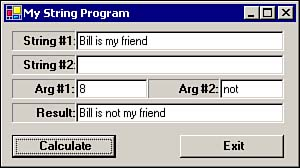For this sample run to work properly, the word not in txtArg2 does have a blank space after it to give the proper spacing in the result string shown in the txtResult text box. Convince yourself that the value of 8 for the first argument produces the proper results.

#### The Replace String Method

The Replace method enables you to replace one substring with another. Again, you could write code on your own to accomplish the same thing, but using Replace is a lot easier than writing the code yourself. (Who needs to reinvent the wheel?)

Remove the current program statements from the btnCalc Click event and add

` Dim buff As String  buff = txtString1.Text txtResult.Text = buff.Replace(txtArg1.Text, txtArg2.Text) `

In this example, the substring held in txtArg1 is replaced with the text held in txtArg2 . A sample run is shown in Figure 6.20.

##### Figure 6.20. Sample run using the Replace method.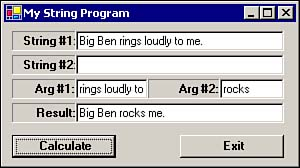The txtString1 text box shows the initial string, whereas txtResult shows the result after the substring has been replaced. In essence, the Replace method locates the first substring, marks its position within the string, deletes the substring characters from the string, and then inserts the replacement string at the position of the original substring. Simple.

As you can see from Figure 6.20, the lengths of the original and replacement substrings do not have to be of equal length. The Replace method is smart enough to take care of the details for us.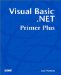Visual Basic .NET Primer Plus
ISBN: 0672324857
EAN: 2147483647
Year: 2003
Pages: 238
Authors: Jack Purdum

Similar book on Amazon